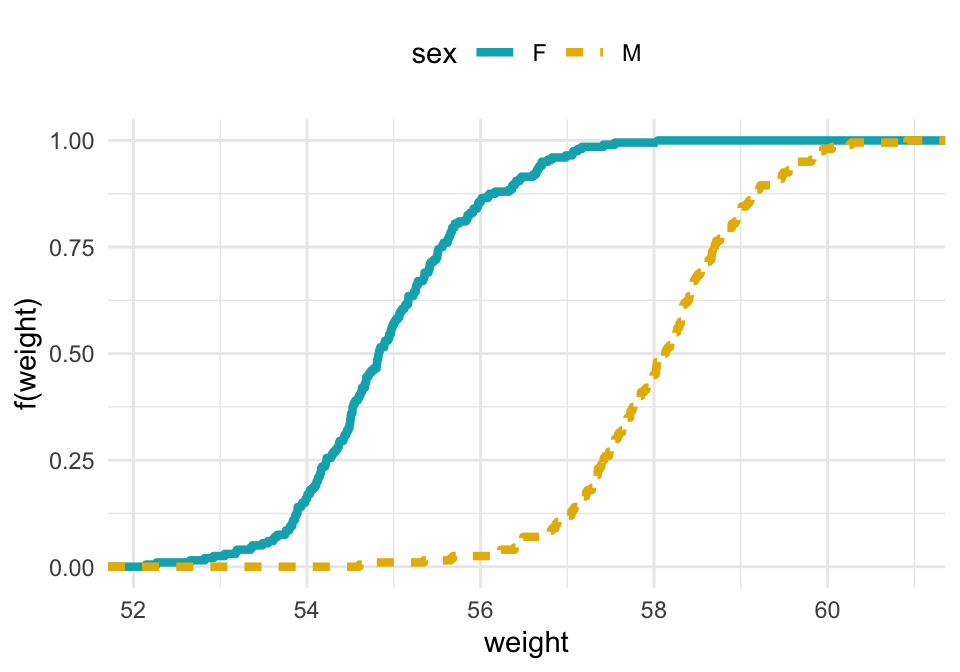# Data Visualization using GGPlot2

## GGPlot ECDF

ECDF (or Empirical cumulative distribution function) provides an alternative visualization of distribution. It reports for any given number the percent of individuals that are below that threshold.

This article describes how to create an ECDF in R using the function stat_ecdf() in ggplot2 package.

Contents:

#### Related Book

GGPlot2 Essentials for Great Data Visualization in R

## Data preparation

Create some data (wdata) containing the weights by sex (M for male; F for female):

set.seed(1234)
wdata = data.frame(
sex = factor(rep(c("F", "M"), each=200)),
weight = c(rnorm(200, 55), rnorm(200, 58))
)

# head(wdata, 4)

Load the ggplot2 package and set the default theme to theme_minimal() with the legend at the top of the plot:

library(ggplot2)
theme_set(
theme_minimal() +
theme(legend.position = "top")
)

## Create ECDF plots

# Another option for geom = "point"
ggplot(wdata, aes(x = weight)) +
stat_ecdf(aes(color = sex,linetype = sex),
geom = "step", size = 1.5) +
scale_color_manual(values = c("#00AFBB", "#E7B800"))+
labs(y = "f(weight)")In the above plots, you can see that:

• about 25% of our females are shorter than 50 inches
• about 50% of males are shorter than 58 inches

## Conclusion

This article shows how to create an ECDF plot using the ggplot2 R package.